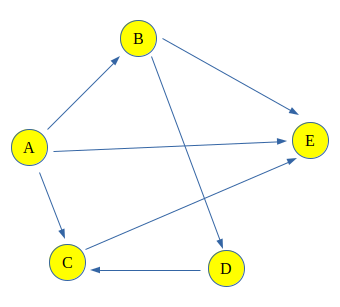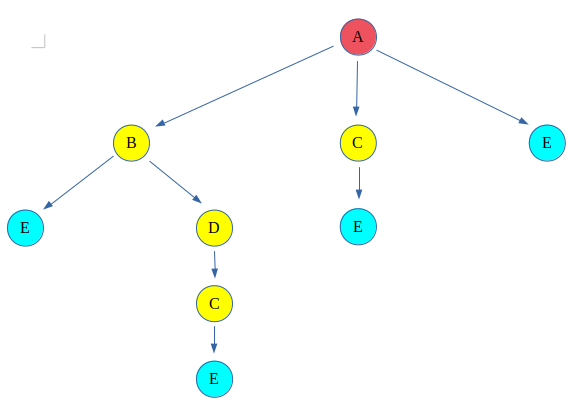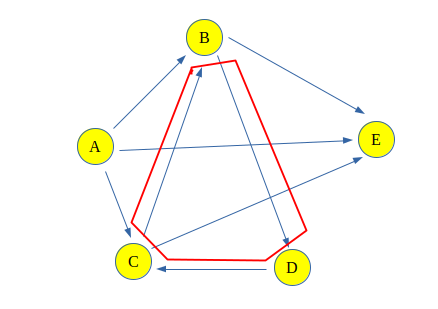GFG App
Open AppBrowser
Continue

# Count all possible Paths between two Vertices

Count the total number of ways or paths that exist between two vertices in a directed graph. These paths don’t contain a cycle, the simple enough reason is that a cycle contains an infinite number of paths and hence they create a problem

Examples:

For the following Graph:Input: Count paths between A and E
Output: Total paths between A and E are 4
Explanation: The 4 paths between A and E are:

A -> E
A -> B -> E
A -> C -> E
A -> B -> D -> C -> E

Input: Count paths between A and C
Output: Total paths between A and C are 2
Explanation: The 2 paths between A and C are:

A -> C
A -> B -> D -> C

## Count paths between two vertices using Backtracking:

To solve the problem follow the below idea:

The problem can be solved using backtracking, which says to take a path and start walking on it and check if it leads us to the destination vertex then count the path and backtrack to take another path. If the path doesn’t lead to the destination vertex, discard the path. This type of graph traversal is called Backtracking.

Backtracking for the above graph can be shown like this:

Note: The red color vertex is the source vertex and the light-blue color vertex is destination, rest are either intermediate or discarded paths.This give four paths between source(A) and destination(E) vertex

## Why this solution will not work for a graph which contains cycles?

The Problem Associated with this is that now if one more edge is added between C and B, it would make a cycle (B -> D -> C -> B). And hence after every cycle through the loop, the length path will increase and that will be considered a different path, and there would be infinitely many paths because of the cycleFollow the given steps to solve the problem:

• Create a recursive function that takes the index of a node of a graph and the destination index. Keep a global or a static variable count to store the count.
• Keep a record of the nodes visited using a visited array and while returning mark the current node to be unvisited to discover other paths.
• If the current node is the destination then increase the count.
• Else for all the adjacent nodes, i.e. nodes that are accessible from the current node, call the recursive function with the index of the adjacent node and the destination.
• Print the Count as the required answer.

Below is the implementation of the above approach.

## C++

 `/*` ` ``* C++ program to count all paths from a source to a` ` ``* destination.` ` ``* https://www.geeksforgeeks.org/count-possible-paths-two-vertices/` ` ``* Note that the original example has been refactored.` ` ``*/` `#include ` `using` `namespace` `std;`   `/*` ` ``* A directed graph using adjacency list representation;` ` ``* every vertex holds a list of all neighbouring vertices` ` ``* that can be reached from it.` ` ``*/` `class` `Graph {` `public``:` `    ``// Construct the graph given the number of vertices...` `    ``Graph(``int` `vertices);` `    ``// Specify an edge between two vertices` `    ``void` `add_edge(``int` `src, ``int` `dst);` `    ``// Call the recursive helper function to count all the` `    ``// paths` `    ``int` `count_paths(``int` `src, ``int` `dst, ``int` `vertices);`   `private``:` `    ``int` `m_vertices;` `    ``list<``int``>* m_neighbours;` `    ``void` `path_counter(``int` `src, ``int` `dst, ``int``& path_count,` `                      ``vector<``bool``>& visited);` `};`   `Graph::Graph(``int` `vertices)` `{` `    ``m_vertices = vertices; ``// unused!!` `    ``/* An array of linked lists - each element corresponds` `    ``to a vertex and will hold a list of neighbours...*/` `    ``m_neighbours = ``new` `list<``int``>[vertices];` `}`   `void` `Graph::add_edge(``int` `src, ``int` `dst)` `{` `    ``m_neighbours[src].push_back(dst);` `}`   `int` `Graph::count_paths(``int` `src, ``int` `dst, ``int` `vertices)` `{` `    ``int` `path_count = 0;` `    ``vector<``bool``> visited(vertices, ``false``);` `    ``path_counter(src, dst, path_count, visited);` `    ``return` `path_count;` `}`   `/*` ` ``* A recursive function that counts all paths from src to` ` ``* dst. Keep track of the count in the parameter.` ` ``*/` `void` `Graph::path_counter(``int` `src, ``int` `dst, ``int``& path_count,` `                         ``vector<``bool``>& visited)` `{` `    ``// If we've reached the destination, then increment` `    ``// count...` `    ``visited[src] = ``true``;` `    ``if` `(src == dst) {` `        ``path_count++;` `    ``}` `    ``// ...otherwise recurse into all neighbours...` `    ``else` `{` `        ``for` `(``auto` `neighbour : m_neighbours[src]) {` `            ``if` `(!visited[neighbour])` `                ``path_counter(neighbour, dst, path_count,` `                             ``visited);` `        ``}` `    ``}` `    ``visited[src] = ``false``;` `}`   `// Driver code` `int` `main()` `{` `    ``// Create a graph given in the above diagram - see link` `    ``Graph g(5);` `    ``g.add_edge(0, 1);` `    ``g.add_edge(0, 2);` `    ``g.add_edge(0, 4);` `    ``g.add_edge(1, 3);` `    ``g.add_edge(1, 4);` `    ``g.add_edge(2, 3);` `    ``g.add_edge(2, 1);` `    ``g.add_edge(3, 2);` `    `  `      ``// Function call` `    ``cout << g.count_paths(0, 4, 5);`   `    ``return` `0;` `}`

## Java

 `// Java program to count all paths from a source` `// to a destination` `import` `java.util.Arrays;` `import` `java.util.Iterator;` `import` `java.util.LinkedList;`   `// This class represents a directed graph using` `// adjacency list representation`   `class` `Graph {`   `    ``// No. of vertices` `    ``private` `int` `V;`   `    ``// Array of lists for` `    ``// Adjacency List` `    ``// Representation` `    ``private` `LinkedList adj[];`   `    ``@SuppressWarnings``(``"unchecked"``) Graph(``int` `v)` `    ``{` `        ``V = v;` `        ``adj = ``new` `LinkedList[v];` `        ``for` `(``int` `i = ``0``; i < v; ++i)` `            ``adj[i] = ``new` `LinkedList<>();` `    ``}`   `    ``// Method to add an edge into the graph` `    ``void` `addEdge(``int` `v, ``int` `w)` `    ``{`   `        ``// Add w to v's list.` `        ``adj[v].add(w);` `    ``}`   `    ``// A recursive method to count` `    ``// all paths from 'u' to 'd'.` `    ``int` `countPathsUtil(``int` `u, ``int` `d, ``int` `pathCount)` `    ``{`   `        ``// If current vertex is same as` `        ``// destination, then increment count` `        ``if` `(u == d) {` `            ``pathCount++;` `        ``}`   `        ``// Recur for all the vertices` `        ``// adjacent to this vertex` `        ``else` `{` `            ``Iterator i = adj[u].listIterator();` `            ``while` `(i.hasNext()) {` `                ``int` `n = i.next();` `                ``pathCount = countPathsUtil(n, d, pathCount);` `            ``}` `        ``}` `        ``return` `pathCount;` `    ``}`   `    ``// Returns count of` `    ``// paths from 's' to 'd'` `    ``int` `countPaths(``int` `s, ``int` `d)` `    ``{`   `        ``// Call the recursive method` `        ``// to count all paths` `        ``int` `pathCount = ``0``;` `        ``pathCount = countPathsUtil(s, d, pathCount);` `        ``return` `pathCount;` `    ``}`   `    ``// Driver Code` `    ``public` `static` `void` `main(String args[])` `    ``{` `        ``Graph g = ``new` `Graph(``5``);` `        ``g.addEdge(``0``, ``1``);` `        ``g.addEdge(``0``, ``2``);` `        ``g.addEdge(``0``, ``3``);` `        ``g.addEdge(``1``, ``3``);` `        ``g.addEdge(``2``, ``3``);` `        ``g.addEdge(``1``, ``4``);` `        ``g.addEdge(``2``, ``4``);`   `        ``int` `s = ``0``, d = ``3``;` `      `  `          ``// Function call` `        ``System.out.println(g.countPaths(s, d));` `    ``}` `}`   `// This code is contributed by shubhamjd.`

## Python3

 `# Python 3 program to count all paths` `# from a source to a destination.`   `# A directed graph using adjacency` `# list representation`     `class` `Graph:`   `    ``def` `__init__(``self``, V):` `        ``self``.V ``=` `V` `        ``self``.adj ``=` `[[] ``for` `i ``in` `range``(V)]`   `    ``def` `addEdge(``self``, u, v):`   `        ``# Add v to u’s list.` `        ``self``.adj[u].append(v)`   `    ``# Returns count of paths from 's' to 'd'` `    ``def` `countPaths(``self``, s, d):`   `        ``# Mark all the vertices` `        ``# as not visited` `        ``visited ``=` `[``False``] ``*` `self``.V`   `        ``# Call the recursive helper` `        ``# function to print all paths` `        ``pathCount ``=` `[``0``]` `        ``self``.countPathsUtil(s, d, visited, pathCount)` `        ``return` `pathCount[``0``]`   `    ``# A recursive function to print all paths` `    ``# from 'u' to 'd'. visited[] keeps track` `    ``# of vertices in current path. path[]` `    ``# stores actual vertices and path_index` `    ``# is current index in path[]` `    ``def` `countPathsUtil(``self``, u, d,` `                       ``visited, pathCount):` `        ``visited[u] ``=` `True`   `        ``# If current vertex is same as` `        ``# destination, then increment count` `        ``if` `(u ``=``=` `d):` `            ``pathCount[``0``] ``+``=` `1`   `        ``# If current vertex is not destination` `        ``else``:`   `            ``# Recur for all the vertices` `            ``# adjacent to current vertex` `            ``i ``=` `0` `            ``while` `i < ``len``(``self``.adj[u]):` `                ``if` `(``not` `visited[``self``.adj[u][i]]):` `                    ``self``.countPathsUtil(``self``.adj[u][i], d,` `                                        ``visited, pathCount)` `                ``i ``+``=` `1`   `        ``visited[u] ``=` `False`     `# Driver Code` `if` `__name__ ``=``=` `'__main__'``:`   `    ``# Create a graph given in the` `    ``# above diagram` `    ``g ``=` `Graph(``4``)` `    ``g.addEdge(``0``, ``1``)` `    ``g.addEdge(``0``, ``2``)` `    ``g.addEdge(``0``, ``3``)` `    ``g.addEdge(``2``, ``0``)` `    ``g.addEdge(``2``, ``1``)` `    ``g.addEdge(``1``, ``3``)`   `    ``s ``=` `2` `    ``d ``=` `3` `    `  `    ``# Function call` `    ``print``(g.countPaths(s, d))`   `# This code is contributed by PranchalK`

## C#

 `// C# program to count all paths from a source` `// to a destination.` `using` `System;` `using` `System.Collections.Generic;`   `// This class represents a directed graph using` `// adjacency list representation` `public` `class` `Graph {`   `    ``// Array of lists for` `    ``// Adjacency List` `    ``// Representation` `    ``private` `List<``int``>[] adj;`   `    ``Graph(``int` `v)` `    ``{` `        ``adj = ``new` `List<``int``>[ v ];` `        ``for` `(``int` `i = 0; i < v; ++i)` `            ``adj[i] = ``new` `List<``int``>();` `    ``}`   `    ``// Method to add an edge into the graph` `    ``void` `addEdge(``int` `v, ``int` `w)` `    ``{`   `        ``// Add w to v's list.` `        ``adj[v].Add(w);` `    ``}`   `    ``// A recursive method to count` `    ``// all paths from 'u' to 'd'.` `    ``int` `countPathsUtil(``int` `u, ``int` `d, ``int` `pathCount)` `    ``{`   `        ``// If current vertex is same as` `        ``// destination, then increment count` `        ``if` `(u == d) {` `            ``pathCount++;` `        ``}`   `        ``// Recur for all the vertices` `        ``// adjacent to this vertex` `        ``else` `{` `            ``foreach``(``int` `i ``in` `adj[u])` `            ``{` `                ``int` `n = i;` `                ``pathCount = countPathsUtil(n, d, pathCount);` `            ``}` `        ``}` `        ``return` `pathCount;` `    ``}`   `    ``// Returns count of` `    ``// paths from 's' to 'd'` `    ``int` `countPaths(``int` `s, ``int` `d)` `    ``{`   `        ``// Call the recursive method` `        ``// to count all paths` `        ``int` `pathCount = 0;` `        ``pathCount = countPathsUtil(s, d, pathCount);` `        ``return` `pathCount;` `    ``}`   `    ``// Driver Code` `    ``public` `static` `void` `Main(String[] args)` `    ``{` `        ``Graph g = ``new` `Graph(5);` `        ``g.addEdge(0, 1);` `        ``g.addEdge(0, 2);` `        ``g.addEdge(0, 3);` `        ``g.addEdge(1, 3);` `        ``g.addEdge(2, 3);` `        ``g.addEdge(1, 4);` `        ``g.addEdge(2, 4);`   `        ``int` `s = 0, d = 3;` `      `  `          ``// Function call` `        ``Console.WriteLine(g.countPaths(s, d));` `    ``}` `}`   `// This code is contributed by Rajput-Ji`

## Javascript

 ``

Output

`3`

Time Complexity: The time complexity of this program is O(2^n), where n is the number of vertices in the graph. This is because in the worst case scenario, the program will have to recursively visit all possible paths from the source to the destination, which can be exponential in the number of vertices.
Auxiliary Space: O(N). Auxiliary stack space used by recursion calls

## Approach 2: Depth-first search (DFS)

#### Step by step explanation:

1. The algorithm starts at the start vertex.
2. The algorithm recursively explores all of the neighbors of the current vertex.
3. If the algorithm reaches the end vertex, it adds the path to a list of paths.
4. The algorithm repeats steps 2 and 3 until it has explored all of the vertices in the graph.

## Python3

 `def` `count_paths(graph, source, destination, visited):` `    ``"""` `    ``Counts the number of paths from the source vertex to the destination vertex in the graph.`   `    ``Args:` `      ``graph: The graph.` `      ``source: The source vertex.` `      ``destination: The destination vertex.` `      ``visited: A set of vertices that have already been visited.`   `    ``Returns:` `      ``The number of paths from the source vertex to the destination vertex.` `    ``"""`   `    ``# If the source vertex is the destination ` `    ``# vertex, then return 1.` `    ``if` `source ``=``=` `destination:` `        ``return` `1`   `    ``# If the source vertex has already ` `    ``# been visited, then return 0.` `    ``if` `source ``in` `visited:` `        ``return` `0`   `    ``# Recursively count the number of paths ` `    ``# from each of the source vertex's ` `    ``# neighbors to the destination vertex.` `    ``count ``=` `0` `    ``for` `neighbor ``in` `graph:` `        ``if` `neighbor ``not` `in` `visited:` `            ``count ``+``=` `count_paths(graph, neighbor,` `                                 ``destination, visited | {source})`   `    ``return` `count`     `# Original inputs` `graph ``=` `{` `    ``0``: [``1``, ``2``, ``4``],` `    ``1``: [``3``, ``4``],` `    ``2``: [``3``, ``1``],` `    ``3``: [``2``]` `}` `source ``=` `0` `destination ``=` `4`   `# Count the number of paths` `count ``=` `count_paths(graph, source, destination, ``set``())`   `# Print the number of paths` `print``(count)`

Output

`3`

Time complexity: O(|V| * |E|), where |V| is the number of vertices in the graph and |E| is the number of edges in the graph.
Auxiliary space: O(|V|), where |V| is the number of vertices in the graph.

My Personal Notes arrow_drop_up